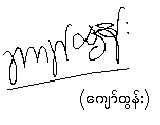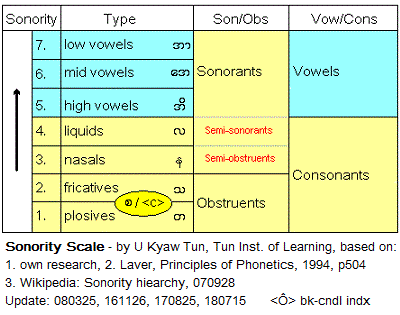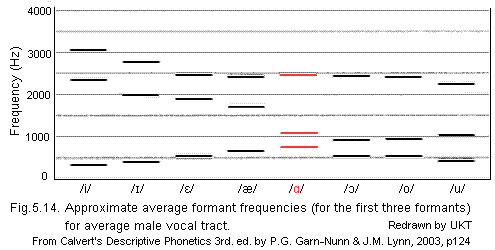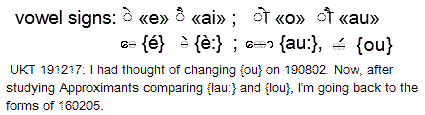Update: 2019-08-07 05:57 PM -0400

#### TIL# Sanskrit Glossary for Buddhists and Hindus : Consonants

SktGlossC1-345.htm

Based on1. Sanskrit Documents ||संस्कृत शब्दार्थ || = स ं स ् क ृ त ,
Write to sanskrit@cheerful.com . 12/22/2018 23:25:17
2. ¤ Student's Pali-English Dictionary, by Maung Tin (name later changed to U Pe Maung Tin), (ref: UPMT-PEDictxxx). Downloaded copies in TIL HD-PDF and SD-PDF libraries:
- UPMT-PaliDict1920<Ô> / Bkp<Ô> (link chk 190114)

Edited by U Kyaw Tun (UKT) (M.S., I.P.S.T., USA), and staff of Tun Institute of Learning (TIL). Not for sale. No copyright. Free for everyone. Prepared for students and staff of TIL  Research Station, Yangon, MYANMAR
- http://www.tuninst.net , www.romabama.blogspot.comCaution: My main difficulty in learning Skt-Dev, is to become familiar with horizontal conjuncts. I've no choice but to rely on my knowledge of Bur-Myan and Pali-Myan, to come up with a compromise in giving the akshara-equivalents, knowing fully well that it would not make my pronunciation of Skt-Dev any better. It might even a detriment. But since, translation is my ultimate aim, using the motto of Shin Kic'si -- the Buddhist grammarian praised by Gautama Buddha himself -- I have to be content with what is available to me.

To aid me in my task, I'm preparing a Sanskrit Glossary for Buddhists and Hindus , based on the Sonority Scale, in which begins with Consonants and Vowels last: an arrangement used in Bur-Myan dictionaries and glossaries:The original Glossary files given by Sanskrit Documents has become so unmanageable in size that I've to divide it into sub-files. Since, I'm including Pali terms from UPMT, I've to subdivide down to columns :

#### This file is on Row #1 Columns 3, 4, & 5

Entries on Skt from SktDoc are in Devanagari characters, and on Pali from U Pe Maung Tin (UPT) are in International Pali characters. Be careful to check diacritics when using UPT. Astrological terms are given for which I'm giving the symbols for:
Luminaries (commonly and mistakenly called Planets): ☼ ☽ ♂ ☿ ♃ ♀ ♄
Rasis (Signs of Zodiac): ♈ ♉ ♊ ♋ ♌ ♍ ♎ ♏ ♐ ♑ ♒ ♓

The 4 pairs below are known as{þa.wuN} "similar or beautiful pairs"{ga.} ग{ga} गा{gi.} गि{gi} गी{gu.} गु{gu} गू{gRRi.} गृThe 2 pairs below are known as{a.þa.wuN} "dissimilar ugly pairs". They are problematical, because some vowels are missing in languages, e.g. /é/ is absent in Eng-Lat, and /ao/ is absent in Bur-Myan but present in Mon-Myan. Because of this fact and also because the back-vowels are very near each other, there are bound to be un-solvable problems phonetically. E.g. /ɑ/, /ɔ/, & /o/. The only way to analyze them is to measure the formants in the speech of modern speakers. Still we do not know how the ancient speakers had pronounced them. I've to compare the "ugly pairs" of different languages and come to a "happy medium" which you can take or ignore. For comparing Skt-Dev to Bur-Myan, I use the diacritics over the individual glyphs.{} गे{gè:} गै ------------- cf.{} के{kè:} कै = क ै{gau:} गो/{gou} गौ --- cf.{kau:} को/{kou} कौ  = क ौ{gRa.} ग्र{gRa} ग्रा
---------------{gRi} ग्री
---------------{gla} : hanging{la.}{Ga.} घ{Ga} घा{GRRi.} घृ{Gau:} घो :  Cf:/{gou} गौ in गौर (gaura) = white{gna.} ङ /{ng} : present in Néwari/Népali

##{ga.} ग

गं (ga.n) = the letter or sound `ga.n
गकारः (gakaaraH) = beginning with the letter `ga'
गगनयात्रिकः (gaganayaatrikaH) = (m) astronaut
गङ्गा (ga.ngaa) = river Ganga
गङ्गासागर (ga.ngaasaagara) = the sea of Ganga (banks of the Ganges)
गच्छताम् (gachchhataam.h) = (may the two)go
गच्छति (gachchhati) = goes
गच्छन् (gachchhan.h) = going
गच्छन्ति (gachchhanti) = they reach
गज (gaja) = Elephant
गजः (gajaH) = elephant
गजकेशरियोग (gajakeshariyoga) = Yoga [Combinations] in which the ☽ Moon is in an angular position (Kendra) or 1,4,7,10 signs from ♃ Jupiter.
Good Yoga for Wealth and Power based on strength and other factors in the chart [Rasi or Bhava charts]
गजेन्द्राणां (gajendraaNaaM) = of lordly elephants
गञ्जीफा (gaJNjiiphaa) = playing cards
गण् (gaN.h) = to count, to consider
गण (gaNa) = Number
गणकऋषिः (gaNakaRishhiH) = the rishi of this stotra is gaNaka
गणना (gaNanaa) = consideration
गणपतये (gaNapataye) = to gaNapati
गणपति (gaNapati) = gaNaanaaM pathiH or lord of groups (of devas)
गणपतिं (gaNapatiM) = Ganesh
गणपतिर्देवता (gaNapatirdevataa) = the god of this stotra
गणपती (gaNapatii) = god of luck and wisdom
गणयति (gaNayati) = (10 up) to count
गणित (gaNita) = mathematics
गणेशविद्या (gaNeshavidyaa) = the knowledge of gaNeshha
गण्ड (gaNDa) = the cheek
गत (gata) = gone
गत( ः) (gata(H)) = (Masc.Nom.S) having gone or the person who has gone
गतं (gataM) = reached (past part.)
गतः (gataH) = returned
गतचिन्ता (gatachintaa) = thinking of
गतरसं (gatarasaM) = tasteless
गतवति (gatavati) = while gone
गतव्यथाः (gatavyathaaH) = freed from all distress
गतसङ्गस्य (gatasa.ngasya) = of one unattached to the modes of material nature
गता (gataa) = became
गताः (gataaH) = having achieved
गतागतं (gataagataM) = death and birth
गतासून् (gataasuun.h) = gata + asuun.h:departed life (dead people)
गति (gati) = the movement
गतिं (gatiM) = progress
गतिः (gatiH) = entrance
गत्वा (gatvaa) = attaining
गदति (gadati) = (1 pp) to say
गन्तव्यं (gantavyaM) = to be reached
गन्तासि (gantaasi) = you shall go
गन्ध (gandha) = smell (masc)
गन्धः (gandhaH) = fragrance
गन्धर्व (gandharva) = of the Gandharvas
गन्धर्वाणां (gandharvaaNaaM) = of the citizens of the Gandharva planet
गन्धान् (gandhaan.h) = smells
गमः (gamaH) = take to
गमन (gamana) = Going
गमनं (gamanaM) = going
गम्यते (gamyate) = one can attain
गरीयः (gariiyaH) = better
गरीयसि (gariiyasi) = (fem.Nom.S) better (thing or person)
गरीयसे (gariiyase) = who are better
गरीयान् (gariiyaan.h) = glorious
गरुड (garuDa) = eagle
गरुडासन (garuDaasana) = the eagle posture

गर्ज् (garj.h) = to thunder
गर्जनम् (garjanam.h) = thundering sound
गर्त (garta) = (m) pit, trench, cavity
गर्दभ (gardabha) = donkey
गर्दभः (gardabhaH) = (m) donkey
गर्भ (garbha) = womb
गर्भं (garbhaM) = pregnancy
गर्भः (garbhaH) = embryo
गर्भपिण्ड (garbhapiNDa) = an embryo
गर्भासन (garbhaasana) = the foetus posture
गर्भिणी (garbhiNii) = (f) a pregnant woman
गर्व (garva) = vanity
गर्वं (garvaM) = arrogance/haughtiness
गल (gala) = neck
गलित (galita) = (adj) dropped off
गलितं (galitaM) = weakened
गवाक्षः (gavaakShaH) = (m) window, an opening for ventilation
गवि (gavi) = in the cow
गहना (gahanaa) = very difficult

##{ga} गा

गां (gaaM) = the planets luminaries
गाङ्ग (gaa.nga) = of the Ganga river
गाण्डीवं (gaaNDiivaM) = the bow of Arjuna
गात्राणि (gaatraaNi) = limbs of the body
गान (gaana) = singing
गायकः (gaayakaH) = (m) singer
गायति (gaayati) = (1 pp) to sing
गायत्री (gaayatrii) = the Gayatri hymns
गायिका (gaayikaa) = (f) singer
गाहते (gaahate) = (1 ap) to plunge
गाहमान (gaahamaana) = (present participle) swimming or floating

##{gi.} गि

गिर् (gir.h) = language
गिरः (giraH) = words
गिरां (giraaM) = of vibrations
गिरि (giri) = mountain
गिरिकन्दर (girikandara) = (neut) ravine
गिरिजा (girijaa) = paarvati
गिरिश् (girish) = God of mountain attributed to Lord Shiva
गिलति (gilati) = to swallow

##{gi} गी

गीत (giita) = (n) song
गीतं (giitaM) = described

##{gu.} गु

गु (gu) = darkness
गुड (guDa) = jaggery
गुडः (guDaH) = (m) jaggery
गुडाकेश (guDaakesha) = O Arjuna
गुडाकेशः (guDaakeshaH) = Arjuna, the master of curbing ignorance
गुडाकेशेन (guDaakeshena) = by Arjuna
गुण (guNa) = qualities
गुणकः (guNakaH) = (m) multiplier, coefficient
गुणकथनपुण्येन (guNakathanapuNyena) = through the merit from praising Thy glories
गुणकर्म (guNakarma) = of works under material influence
गुणकर्मसु (guNakarmasu) = in material activities
गुणतः (guNataH) = by the modes of material nature
गुणत्रयः (guNatrayaH) = three qualities i.e satvaH , rajaH \tamaH
गुणन् (guNan.h) = praising
गुणनम् (guNanam.h) = (n) multiplication
गुणनिधिं (guNanidhiM) = the stock-pile of good qualities
गुणभेदतः (guNabhedataH) = in terms of different modes of material nature
गुणभोक्तृ (guNabhoktRi) = master of the gunas
गुणमयी (guNamayii) = consisting of the three modes of material nature
गुणमयैः (guNamayaiH) = consisting of the gunas
गुणवान् (guNavaan.h) = a man with good qualities
गुणसंख्याने (guNasa.nkhyaane) = in terms of different modes
गुणसङ्गः (guNasa.ngaH) = the association with the modes of nature
गुणज्ञ (guNaGYa) = one who knows qualities (one who is a patron of good qualities)
गुणांक (guNaa.nka) = coefficient
गुणाः (guNaaH) = senses
गुणाङ्क (guNaa.nka) = coefficient
गुणाङ्क (guNaa.nka) = coefficient
गुणातीतः (guNaatiitaH) = transcendental to the material modes of nature
गुणान् (guNaan.h) = the three modes of nature
गुणान्वितं (guNaanvitaM) = under the spell of the modes of material nature
गुणेभ्यः (guNebhyaH) = to the modes of nature
गुणेषु (guNeshhu) = in sense gratification
गुणैः (guNaiH) = by the qualities
गुणोत्तर (guNottara) = ratio
गुनवत् (gunavat.h) = man with good qualities
गुप् (gup.h) = guard, hide
गुप्त (gupta) = secret
गुप्तासन (guptaasana) = the hidden posture
गुरु (guru) = teacher
गुरुः (guruH) = teacher; weighty
गुरुचरणाम्बुज (gurucharaNaambuja) = the lotus feet of the teacher/guru
गुरुणापि (guruNaapi) = even though very difficult
गुरुत्वं (gurutvaM) = (abstract noun)greatness;weeightiness
गुरुत्वाअकर्षण (gurutvaaakarshhaNa) = gravitational attraction
गुरुन् (gurun.h) = the superiors
गुरुवार (guruvaara) = Thrusday
गुरू (guruu) = teacher, preceptor, long syllables as against short(laghuu)
गुलिका (gulikaa) = (f) tablet, pill
गुल्म (gulma) = the spleen
गुहा (guhaa) = (fem) cave
गुह्यं (guhyaM) = confidential subject
गुह्यतमं (guhyatamaM) = the most confidential
गुह्यतरं (guhyataraM) = still more confidential
गुह्यात् (guhyaat.h) = than confidential
गुह्यानां (guhyaanaaM) = of secrets

##{gu} गू

गूढ (guuDha) = (adj) secret, mysterious

##{gRRi.} गृ

गृञ्जनकम् (gRiJNjanakam.h) = (n) carrot
गृणन्ति (gRiNanti) = are offering prayers
गृणामि (gRiNaami) = I hold
गृध्रः (gRidhraH) = (m) eagle
गृह (gRiha) = house
गृहगोधिका (gRihagodhikaa) = (f) house lizard
गृहपाठः (gRihapaaThaH) = (m) homework
गृहस्थाः (gRihasthaaH) = householders
गृहिणः (gRihiNaH) = (Masc.Nom.pl.) house-holders
गृहीत्वा (gRihiitvaa) = holding the
गृह्णन् (gRihNan.h) = accepting
गृह्णाति (gRihNaati) = to catch
गृह्यते (gRihyate) = can be so controlled

##{gé} गे

गेय (geya) = that which can be sung
गेयं (geyaM) = is to be sung
गेहे (gehe) = in the house

##{gau:} गो

गो (go) = cow / bull
गोओष्ठी (gooshhThii) = (f) seminar
गोत्र (gotra) = family, race
गोमुख (gomukha) = musical instrument resembling a cow's face
गोमुखाः (gomukhaaH) = horns
गोमुखासन (gomukhaasana) = the cow-faced posture
गोरक्षासन (gorakShaasana) = the cowherd posture
गोविन्द (govinda) = Lord KRishna
गोविन्दं (govindaM) = Govinda

##/{gou} गौ

गौर (gaura) = white
गौरव (gaurava) = glory
गौरवं (gauravaM) = respect

##{gRa.} ग्र

ग्रन्थालयः (granthaalayaH) = (m) library
ग्रन्थिः (granthiH) = (m) gland
ग्रन्थी (granthii) = a knot, obstruction in the chitrini
ग्रसते (grasate) = (1 pp) to swallow
ग्रसमानः (grasamaanaH) = devouring
ग्रसिष्णु (grasishhNu) = devouring
ग्रस्तं (grastaM) = having been caught/seized
ग्रह (graha) = astro Planet Luminary [UKT 190730: Astrologically, it is one of the gods ruling the luminous world,
which travels across the sky, who controls (or "grabs") the human who has come under his control.

##{gRa} ग्रा

ग्रामम् (graamam.h) = (n) village
ग्राहकः (graahakaH) = (m) customer
ग्राहय (graahaya) = (verbal stem) to cause to be caught
ग्राहान् (graahaan.h) = things
ग्राहेण (graaheNa) = with endeavour
ग्राह्य (graahya) = what is to be grasped
ग्राह्यं (graahyaM) = accessible

##{gRi} ग्री

ग्रीवं (griivaM) = neck

##{gla} : hanging{la.}

ग्लानिः (glaaniH) = discrepancies
ग्लायति (glaayati) = (1 pp) to fade

##{Ga.} घ

घट (ghaTa) = pot
घटः (ghaTaH) = (m) earthen pot
घटक (ghaTaka) = component
घटि (ghaTi) = Hour
घतः (ghataH) = (m) earthen pot
घन (ghana) = dark, ghana also means cloud
घनता (ghanataa) = density
घनसम (ghanasama) = Like cloud
घनिष (ghanishha) = well-built

##{Ga} घा

घातयति (ghaatayati) = causes to hurt
घातुकः (ghaatukaH) = (m) butcher

##{GRRi.} घृ

घृणा (ghRiNaa) = (f) hatred
घृत (ghRita) = purified butter, Hindi ghee
घृतं (ghRitaM) = (Nr.nom. + acc. S) ghee; clarified butter

##{Gau:} घो

Cf:/{gou} गौ in गौर (gaura) = white

घोरं (ghoraM) = horribleघोरे (ghore) = ghastly
घोष (ghoshha) = noise
घोषः (ghoshhaH) = vibration
घोषयति (ghoshhayati) = (10 up) to proclaim, announce

घ्नतः (ghnataH) = being killed
घ्नी (ghnii) = destroying

घ्राणं (ghraaNaM) = smelling power